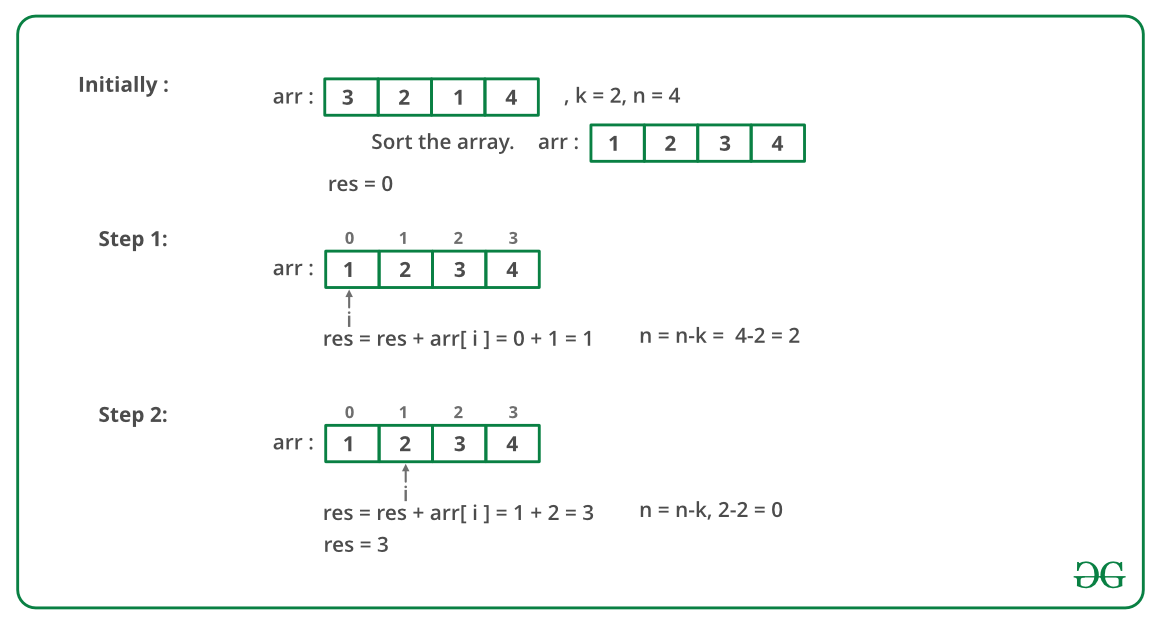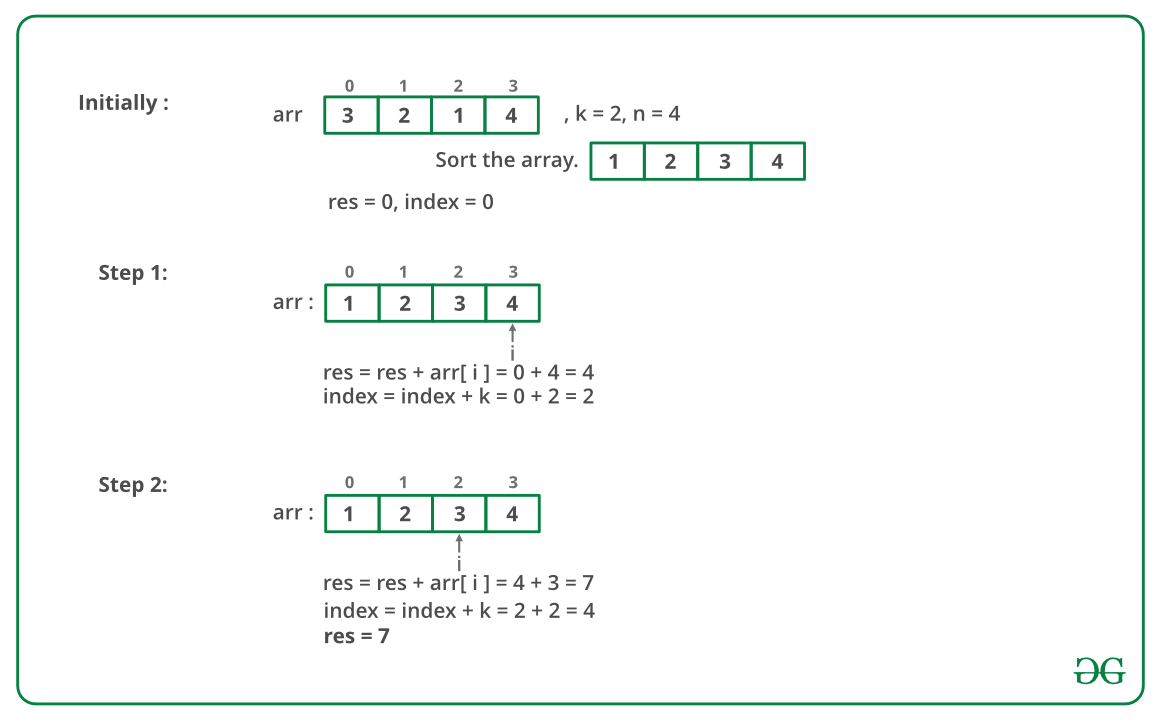Open in App
Not now

# Find the minimum and maximum amount to buy all N candies

• Difficulty Level : Easy
• Last Updated : 24 Jan, 2023

In a candy store, there are N different types of candies available and the prices of all the N different types of candies are provided. There is also an attractive offer by the candy store. We can buy a single candy from the store and get at most K other candies (all are different types) for free.

1. Find the minimum amount of money we have to spend to buy all the N different candies.
2. Find the maximum amount of money we have to spend to buy all the N different candies.

In both cases, we must utilize the offer and get the maximum possible candies back. If k or more candies are available, we must take k candies for every candy purchase. If less than k candies are available, we must take all candies for a candy purchase.

Examples:

```Input :
price[] = {3, 2, 1, 4}
k = 2
Output :
Min = 3, Max = 7
Explanation :
Since k is 2, if we buy one candy we can take
So in the first case we buy the candy which
costs 1 and take candies worth 3 and 4 for
free, also you buy candy worth 2 as well.
So min cost = 1 + 2 = 3.
In the second case we buy the candy which
costs 4 and take candies worth 1 and 2 for
free, also We buy candy worth 3 as well.
So max cost = 3 + 4 = 7.```
Recommended Practice

One important thing to note is, we must use the offer and get maximum candies back for every candy purchase. So if we want to minimize the money, we must buy candies at minimum cost and get candies of maximum costs for free. To maximize the money, we must do the reverse. Below is an algorithm based on this.

```First Sort the price array.

For finding minimum amount :
and reduce k free candies from last with
every single purchase.

For finding maximum amount :
Start purchasing candies from the end
and reduce k free candies from starting
in every single purchase.```

Below image is an illustration of the above approach:

Minimum amount :Maximum amount :Below is the implementation of the above approach:

## C++

 `// C++ implementation to find the minimum` `// and maximum amount` `#include ` `using` `namespace` `std;`   `// Function to find the minimum amount` `// to buy all candies` `int` `findMinimum(``int` `arr[], ``int` `n, ``int` `k)` `{` `    ``int` `res = 0;` `    ``for` `(``int` `i = 0; i < n; i++) {` `        ``// Buy current candy` `        ``res += arr[i];`   `        ``// And take k candies for free` `        ``// from the last` `        ``n = n - k;` `    ``}` `    ``return` `res;` `}`   `// Function to find the maximum amount` `// to buy all candies` `int` `findMaximum(``int` `arr[], ``int` `n, ``int` `k)` `{` `    ``int` `res = 0, index = 0;`   `    ``for` `(``int` `i = n - 1; i >= index; i--) ` `    ``{` `        ``// Buy candy with maximum amount` `        ``res += arr[i];`   `        ``// And get k candies for free from` `        ``// the starting` `        ``index += k;` `    ``}` `    ``return` `res;` `}`   `// Driver code` `int` `main()` `{` `    ``int` `arr[] = { 3, 2, 1, 4 };` `    ``int` `n = ``sizeof``(arr) / ``sizeof``(arr);` `    ``int` `k = 2;` `    ``sort(arr, arr + n);`   `    ``// Function call` `    ``cout << findMinimum(arr, n, k) << ``" "` `         ``<< findMaximum(arr, n, k) << endl;` `    ``return` `0;` `}`

## Java

 `// Java implementation to find the` `// minimum and maximum amount` `import` `java.util.*;`   `class` `GFG {`   `    ``// Function to find the minimum` `    ``// amount to buy all candies` `    ``static` `int` `findMinimum(``int` `arr[], ``int` `n, ``int` `k)` `    ``{` `        ``int` `res = ``0``;` `        ``for` `(``int` `i = ``0``; i < n; i++) {` `            ``// Buy current candy` `            ``res += arr[i];`   `            ``// And take k candies for free` `            ``// from the last` `            ``n = n - k;` `        ``}` `        ``return` `res;` `    ``}`   `    ``// Function to find the maximum` `    ``// amount to buy all candies` `    ``static` `int` `findMaximum(``int` `arr[], ``int` `n, ``int` `k)` `    ``{` `        ``int` `res = ``0``, index = ``0``;`   `        ``for` `(``int` `i = n - ``1``; i >= index; i--) ` `        ``{` `            ``// Buy candy with maximum amount` `            ``res += arr[i];`   `            ``// And get k candies for free from` `            ``// the starting` `            ``index += k;` `        ``}` `        ``return` `res;` `    ``}`   `    ``// Driver code` `    ``public` `static` `void` `main(String[] args)` `    ``{` `        ``int` `arr[] = { ``3``, ``2``, ``1``, ``4` `};` `        ``int` `n = arr.length;` `        ``int` `k = ``2``;` `        ``Arrays.sort(arr);`   `        ``// Function call` `        ``System.out.println(findMinimum(arr, n, k) + ``" "` `                           ``+ findMaximum(arr, n, k));` `    ``}` `}`   `// This code is contributed by prerna saini`

## Python3

 `# Python implementation` `# to find the minimum` `# and maximum amount`   `# Function to find` `# the minimum amount` `# to buy all candies`     `def` `findMinimum(arr, n, k):`   `    ``res ``=` `0` `    ``i ``=` `0` `    ``while``(i``=` `index):`   `        ``# Buy candy with` `        ``# maximum amount` `        ``res ``+``=` `arr[i]`   `        ``# And get k candies` `        ``# for free from` `        ``# the starting` `        ``index ``+``=` `k` `        ``i ``-``=` `1`   `    ``return` `res`   `# Driver code` `arr ``=` `[``1``,``2``,``3``,``4``,``5``,``6``,``7``,``8``,``9``,``10``]` `n ``=` `len``(arr)` `k ``=` `0`   `arr.sort()`   `# Function call` `print``(findMinimum(arr, n, k), ``" "``,` `      ``findMaximum(arr, n, k))`   `# This code is contributed` `# by Anant Agarwal.`

## C#

 `// C# implementation to find the` `// minimum and maximum amount` `using` `System;`   `public` `class` `GFG {`   `    ``// Function to find the minimum` `    ``// amount to buy all candies` `    ``static` `int` `findMinimum(``int``[] arr, ``int` `n, ``int` `k)` `    ``{` `        ``int` `res = 0;` `        ``for` `(``int` `i = 0; i < n; i++) ` `        ``{`   `            ``// Buy current candy` `            ``res += arr[i];`   `            ``// And take k candies for` `            ``// free from the last` `            ``n = n - k;` `        ``}`   `        ``return` `res;` `    ``}`   `    ``// Function to find the maximum` `    ``// amount to buy all candies` `    ``static` `int` `findMaximum(``int``[] arr, ``int` `n, ``int` `k)` `    ``{` `        ``int` `res = 0, index = 0;`   `        ``for` `(``int` `i = n - 1; i >= index; i--) ` `        ``{` `            ``// Buy candy with maximum` `            ``// amount` `            ``res += arr[i];`   `            ``// And get k candies for free` `            ``// from the starting` `            ``index += k;` `        ``}`   `        ``return` `res;` `    ``}`   `    ``// Driver code` `    ``public` `static` `void` `Main()` `    ``{` `        ``int``[] arr = { 3, 2, 1, 4 };` `        ``int` `n = arr.Length;` `        ``int` `k = 2;` `        ``Array.Sort(arr);`   `        ``// Function call` `        ``Console.WriteLine(findMinimum(arr, n, k) + ``" "` `                          ``+ findMaximum(arr, n, k));` `    ``}` `}`   `// This code is contributed by Sam007.`

## PHP

 `= ``\$index``; ``\$i``--)` `    ``{` `        `  `        ``// Buy candy with maximum amount` `        ``\$res` `+= ``\$arr``[``\$i``];`   `        ``// And get k candies` `        ``// for free from` `        ``// the starting` `        ``\$index` `+= ``\$k``;` `    ``}` `    ``return` `\$res``;` `}`   `    ``// Driver Code` `    ``\$arr` `= ``array``(3, 2, 1, 4);` `    ``\$n` `= sizeof(``\$arr``);` `    ``\$k` `= 2;` `    ``sort(``\$arr``); sort(``\$arr``,``\$n``);`   `    ``// Function call` `    ``echo` `findMinimum(``\$arr``, ``\$n``, ``\$k``),``" "` `            ``,findMaximum(``\$arr``, ``\$n``, ``\$k``);` `    ``return` `0;`   `// This code is contributed by nitin mittal.` `?>`

## Javascript

 ``

Output

`3 7`

Time Complexity : O(nlogn)
Auxiliary Space: O(1)

Another Implementation:
We can use the help of The Least integer function (Ceiling function) using built-in ceil() function to implement:

Below is the implementation in Python:

## C++

 `// C++ implementation` `// to find the minimum` `// and maximum amount` `#include ` `using` `namespace` `std;`   `// function to find the maximum` `// and the minimum cost required` `void` `find(vector<``int``> arr, ``int` `n, ``int` `k)` `{`   `    ``// Sort the array` `    ``sort(arr.begin(), arr.end());` `    ``int` `b = ``ceil``(n / k * 1.0);` `    ``int` `min_sum = 0, max_sum = 0;`   `    ``for``(``int` `i = 0; i < b; i++) ` `      ``min_sum += arr[i];` `    ``for``(``int` `i = 2; i < arr.size(); i++)` `      ``max_sum += arr[i];`   `    ``// print the minimum cost` `    ``cout << ``"minimum "` `<< min_sum << endl;`   `    ``// print the maximum cost` `    ``cout << ``"maximum "` `<< max_sum << endl;`   `}`     `// Driver code` `int` `main()` `{` `  ``vector<``int``> arr = {3, 2, 1, 4};` `  ``int` `n = arr.size();` `  ``int` `k = 2;`   `  ``// Function call` `  ``find(arr,n,k);` `}`   `// This code is contributed by mohit kumar 29.`

## Java

 `// Java implementation to find the minimum` `// and maximum amount` `import` `java.io.*;` `import` `java.util.Arrays;` `import` `java.lang.Math;`   `class` `GFG{`   `// Function to find the maximum` `// and the minimum cost required` `static` `void` `find(``int``[] arr, ``int` `n, ``int` `k)` `{` `    `  `    ``// Sort the array` `    ``Arrays.sort(arr);` `    ``int` `b = (``int``)Math.ceil(n / k * ``1.0``);` `    ``int` `min_sum = ``0``, max_sum = ``0``;`   `    ``for``(``int` `i = ``0``; i < b; i++)` `        ``min_sum += arr[i];` `    ``for``(``int` `i = ``2``; i < arr.length; i++)` `        ``max_sum += arr[i];`   `    ``// Print the minimum cost` `    ``System.out.println(``"minimum "` `+ min_sum);`   `    ``// Print the maximum cost` `    ``System.out.println(``"maximum "` `+ max_sum);` `}`   `// Driver code` `public` `static` `void` `main (String[] args)` `{` `    ``int``[] arr = { ``3``, ``2``, ``1``, ``4` `};` `    ``int` `n = arr.length;` `    ``int` `k = ``2``;`   `    ``// Function call` `    ``find(arr, n, k);` `}` `}`   `// This code is contributed by shivanisinghss2110`

## Python3

 `# Python implementation` `# to find the minimum` `# and maximum amount`   `#import ceil function` `from` `math ``import` `ceil`   `# function to find the maximum` `# and the minimum cost required` `def` `find(arr,n,k): ` `    `  `    ``# Sort the array` `    ``arr.sort()` `    ``b ``=` `int``(ceil(n``/``k))` `    `  `    ``# print the minimum cost` `    ``print``(``"minimum "``,``sum``(arr[:b]))` `    `  `    ``# print the maximum cost` `    ``print``(``"maximum "``, ``sum``(arr[``-``b:]))` `    `  `    `  `# Driver Code` `arr ``=` `[``3``, ``2``, ``1``, ``4``]` `n ``=` `len``(arr)` `k ``=` `2`   `# Function call` `find(arr,n,k)`

## C#

 `// C# implementation to find the minimum` `// and maximum amount` `using` `System;`   `class` `GFG{`   `// Function to find the maximum` `// and the minimum cost required` `static` `void` `find(``int``[] arr, ``int` `n, ``int` `k)` `{` `    `  `    ``// Sort the array` `    ``Array.Sort(arr);` `    ``int` `b = (``int``)Math.Ceiling(n / k * 1.0);` `    ``int` `min_sum = 0, max_sum = 0;`   `    ``for``(``int` `i = 0; i < b; i++)` `        ``min_sum += arr[i];` `    ``for``(``int` `i = 2; i < arr.Length; i++)` `        ``max_sum += arr[i];`   `    ``// Print the minimum cost` `    ``Console.WriteLine(``"minimum "` `+ min_sum);`   `    ``// Print the maximum cost` `    ``Console.WriteLine(``"maximum "` `+ max_sum);` `}`   `// Driver code` `public` `static` `void` `Main()` `{` `    ``int``[] arr = { 3, 2, 1, 4 };` `    ``int` `n = arr.Length;` `    ``int` `k = 2;`   `    ``// Function call` `    ``find(arr, n, k);` `}` `}`   `// This code is contributed by ukasp`

## Javascript

 ``

Output

```('minimum ', 3)
('maximum ', 7)```

Time Complexity: O(nlog(n))
Auxiliary Space: O(1)

This article is contributed by Sahil Chhabra. If you like GeeksforGeeks and would like to contribute, you can also write an article using write.geeksforgeeks.org or mail your article to contribute@geeksforgeeks.org. See your article appearing on the GeeksforGeeks main page and help other Geeks.